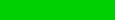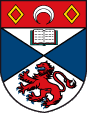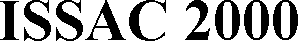### Tutorials

### Date and Location

Tutorials will take place on August 6, 2000, immediately preceding the ISSAC 2000 conference, and they will be held at St Andrews University.

Spaces in the tutorials are limited and will be assigned in the order that registrations are received. A tutorial will be held only if a minimum number of registrations is received. If a tutorial must be cancelled, registrants will be informed by July 18. Please refer to our cancellation and refund policy for more information.

### Schedule

Tutorial 1: Martin Escardo, "Exact Numerical Computation", 9:00-12:00. View abstract.

Tutorial 2: Alexander Hulpke, "Using GAP", 13:00-16:00. View abstract.

Tutorial 3: Mohamed Omar Rayes, "Application of Computer Algebra in Mathematics Education", 16:30-19:30. Sponsored by Texas Instruments. View abstract.

### Fees

Please note that registration is required for all tutorials.

Tutorial 1 - 31 GBP (student rate: 22 GBP)
Tutorial 2 - 31 GBP (student rate: 22 GBP)
Tutorials 1 and 2 - 56 GBP (student rate: 38 GBP)
Tutorial 3 - free (sponsored by Texas Instruments); registration is required

### Abstracts

#### Tutorial 1: Exact Numerical Computation

This tutorial will present the theory and practice of exact numerical computation.

The usual approach to numerical computation consists of approximating the set of real numbers by a large but finite set of real numbers, usually floating-point numbers. It is well-known that, in the absence of careful numerical analysis, the obtained results tend to be unreliable due to the introduction, propagation and accumulation of round-off errors.

A simple example is given by iterates of the logistic map f(x)=4x(1-x). Starting from, say, 0.671875, simple and double precision computations in the IEEE standard produce 0.934518 and 0.757154 as values of the 60th iterate, while the correct value rounded to six decimal digits is 0.315445.

In commercially available computer algebra systems, one can specify an arbitrarily large precision for numerical computations. But, once chosen, the precision is fixed, and round-off errors still occur. It is usually (but not always!) the case that a sufficiently large precision guarantees that the results will be correct to within a specified degree of accuracy. However, it is difficult or unfeasible to predict the necessary precision in advance. Moreover, the precision may be unacceptably large.

Instead of approximating a number by a fixed-length finite numeral, one can realize a number by a "generator". A real number generator is a procedure that successively generates as many digits as one is willing to wait for (and, of course, as the resources of the computer allow). Thus, operations on numbers are realized by operations on number generators. For instance, addition is realized by a process that, given generators for numbers x and y, produces a generator for the number x+y. In this case, computations are usually performed by demand; if one wants 5 correct digits of y=g(f(x)), one may need 20 digits of the intermediate computation f(x), and 7 digits of the input x. In this way, round-off errors are completely avoided.

Exact numerical computation on the reals via potentially infinite representations was proposed by Wiedmer in 1980 and further investigated by a number of authors, including Boehm, Cartwright and Vuillemin. But the idea goes back to constructive mathematics, with the work of Brouwer in 1920 and Bishop in 1967, and recursion theory, with the work of Turing, Rice, Grzegorczyk, Kleene, Vesley, Pour-el, Richards, Weihrauch and Kreitz, among others.

In constructive analysis, the trichotomy law "x<a or x=a or x>a" fails. This is reflected in the fact that the (in)equality relations are undecidable when numbers are presented by generators. This is usually overcome by the constructive law "a<b => x<b or x>a". From a practical point of view, this means that algorithms for finite-precision arithmetic usually don't give rise to algorithms for potentially-infinite-precision arithmetic by a simple change of underlying representation of numbers.

#### Tutorial 2: Using GAP

The aim of this tutorial is to give an introduction to the system GAP for mathematicians and computer scientists who want to use it in their own research, be it as a basis for implementations, or to just utilize the built-in functionality.

GAP is a free system for computational discrete mathematics which has found use in many areas beyond its initial inception as system for group theory.

Its interpretative nature and convenient list functions make it a comfortable environment for prototyping, while the implementation of time-critical subroutines in the C-language kernel maintains a satisfactory performance.

Of particular interest might be its type system, which does not enforce rigid types, but aims to reflect the known mathematical (and implementational) properties of objects and seems to suit computer algebra very well.

Topics include:

• The basic types.
• How standard mathematical concepts are implemented in the system, and how to phrase problems in a suitable way for the system.
• The type system and method selection.
• Creating new objects and extending the system.
• Use of Data Libraries.

The tutorial will only assume prior usage of a computer and knowledge of (any) programming language, it will not assume familiarity with any particular area of mathematics.

#### Tutorial 3: Application of Computer Algebra in Mathematics EducationSponsored by Texas Instruments

Mohamed Omar Rayes
mrayes@ti.com

The aim of the tutorial is to provide an interdisciplinary forum for researchers and teachers in education to present, discuss, and exchange experiences in the use of symbolic algebra in mathematics education. Also, the aim of this tutorial is to provide a platform for researchers and developers of computer algebra tools to exchange ideas and information in their latest development.

Abstracts for individual presentations are available here.

### Tutorials Chair

Josef Schicho
Research Institute for Symbolic Computation (RISC-Linz)
Johannes Kepler University
A-4232 Schloss Hagenberg, Austria
Phone: +43 732 2468 9961
Fax: +43 732 2468 9930
Josef.Schicho@risc.uni-linz.ac.at

[Home Page] - [Dates] - [Program] - [Papers] - [Posters] - [Tutorials] - [Registration] - [Committee] - [Sponsors] - [St Andrews University] - [Travel] - [Accommodation] - [ISSAC Organization]

P. Chin